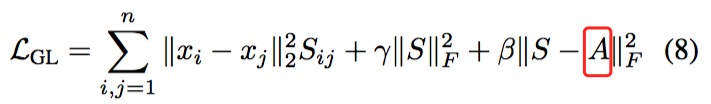# 图模块

PICK: Processing Key Information Extraction from Documents using Improved Graph Learning-Convolutional Networks

# 先导知识

## GLCN

PICK用的图卷积，参考的是Jiang Bo的《GLCN：Semi-supervised learning with graph learning-convolutional networks 2019》，2，这个网络是为了。。。todo

GLCN的主要优点是能将给定的标签和估计的标签合并在一起，因此可以提供有用的“弱”监督信息来改进（或学习）图的结构，并且便于在未知标签估计中使用图卷积运算。 作者提出的GLCN的一个目的是通过集成图学习和图卷积，希望能通过半监督的方式学习到最优的图结构，以用于后续的相关任务。GLCN主要是两块：图学习、图卷积。

【A、图学习】• $x_i$维度为$\mathbb R^{n \times p}$
• $a^T$维度为$\mathbb R^p$For some problems, when an initial graph A is available, we can incorporate it in our graph learning【B、图卷积】+=>2) 图卷积

$\alpha_{ij}^0 = W^0_{\alpha} [ x_{ij},y_{ij},\frac{w_i}{h_i},\frac{h_j}{h_i},\frac{w_j}{h_i},\frac{T_j}{T_i} ]$

# PICK的思路PICK网络，整体上来说，就是4步：

1、做Transformer，得到一个框内文字的语义表达

2、做CNN，得到一个框的图像表达

3、合并1、2的表达，然后用它们，组成一个图，通过GLCN来学些节点（框）的关系 => 邻接矩阵

4、通过有边、顶点的原始表达（1、2表达的合并），然后通过考虑其他特性 $\alpha(6维)$，一起进行图卷积，学出一个图节点们的隐含表达$h_i$。

5、然后把一个框的表达，也就是上面说的“原始表达”（1、2表达的合并），

1、Transformer的输入是每个句子，每个字都是一个word2vec（字的vector吗？），所以输入是：$[T,D_1]$；输出是经过transformer学习后的$[T,D_2]$

2、CNN，就每个小bbox的图片$[H,W,3]$，过这个CNN，然后得到一个，$[H’,W’,D_1]$，但是貌似论文做了一个resize成$[H’‘,W’‘,D_1]，且, H’’*W’‘=T$，哈，诡异哈？！我理解，就是为了后面和1中的Transformer的结果做element-wise add用。

3、然后你从$1 \odot 2$的bboxes们中，抽样出一个图？为何要抽样？为何不用完全的一张图？！不知，没懂。反正你就理解，丫是一张图。这张图的节点都是光秃秃的，没有边的关系，每个点上，也就是每个bbox，有个$1 \odot 2$合体的embeding表示：$[N’,T,D_1]$，$N’$是抽样出来的数量。

4、然后你要通过GLCN来进行“Graph Learning”，来学习节点间是不是有关系了？怎么学？就是算2个节点之间的距离。2个节点的值是固定的，不变的。是从$1 \odot 2$中得到的那个element-wise add